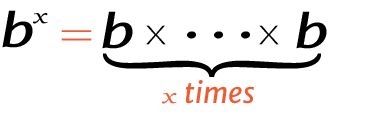# 79 raise to the power of 3

Place the base and exponent in the power calculator to get the right answer for value of 3^79, 379 (3 power 79), or 79 raised to the power of 3.

## How to calculate 3 raise to the power of 79?

This is how you can solve (3) 79 manually.

Solution:

Step 1: Simplify the data.

Base
ExponentStep 2: Multiply the 3 to itself 79 times.

Using this exponents calculator could be much easier to figure out an exponential expression.